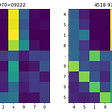# What is Variational Inference?

In the simplest of terms, variational inference is a method of approximating a complex distribution with some family of parameterized distributions.

# Why do we need it?

Let's say we have some observed data X and some unobserved(latent) variable z(what the latent variable is used for doesn't matter much for now). Using full Bayesian inference we can then compute the posterior distribution of z given the data X as:

In most interesting applications, p(X|z) is modelled using a neural network, thereby making the computation of the denominator in Figure 1 intractable. Variational inference helps in sidestepping this issue by approximating the posterior distribution p(z|X) with some simpler distribution q(z) which is “close” to our target distribution. The approximate distribution is chosen from a family of distributions Q.

# How do you do variational inference?

Since our goal is to find a distribution which is “close” to the true posterior we will need to have some sort of metric that can quantify the closeness. The KL-divergence between two distribution is one such metric. Given two distributions with the same domain, the KL divergence is given by:

Note that the KL divergence is always greater than or equal to zero and achieve its minimum value when q(z) = p(z).

Given such a metric, finding an approximation to the posterior distribution p(z|X) can be achieved by solving the following optimization problem.

This, however, is a hard optimization problem to solve. To see why let's unfold the KL term,

Form the above equations its can be observed that estimating the KL term involved computing p(x) which is hard. Luckily, since we only need to minimize the KL, we can optimize another objective, the ELBO, given by

The ELBO is equivalent to the negative KL + log p(x). Thus maximizing the ELBO is the same as minimizing the KL divergence.

--

--

--

## More from Mohammed Suhail

Website: mohammedsuhail.net

Love podcasts or audiobooks? Learn on the go with our new app.## Mohammed Suhail

Website: mohammedsuhail.net

## Can Transformers learn to add like humans?## What is Non-Negative Matrix Factorization (NMF)?## Explainable AI: Shapley Values and the Best Bowling Team